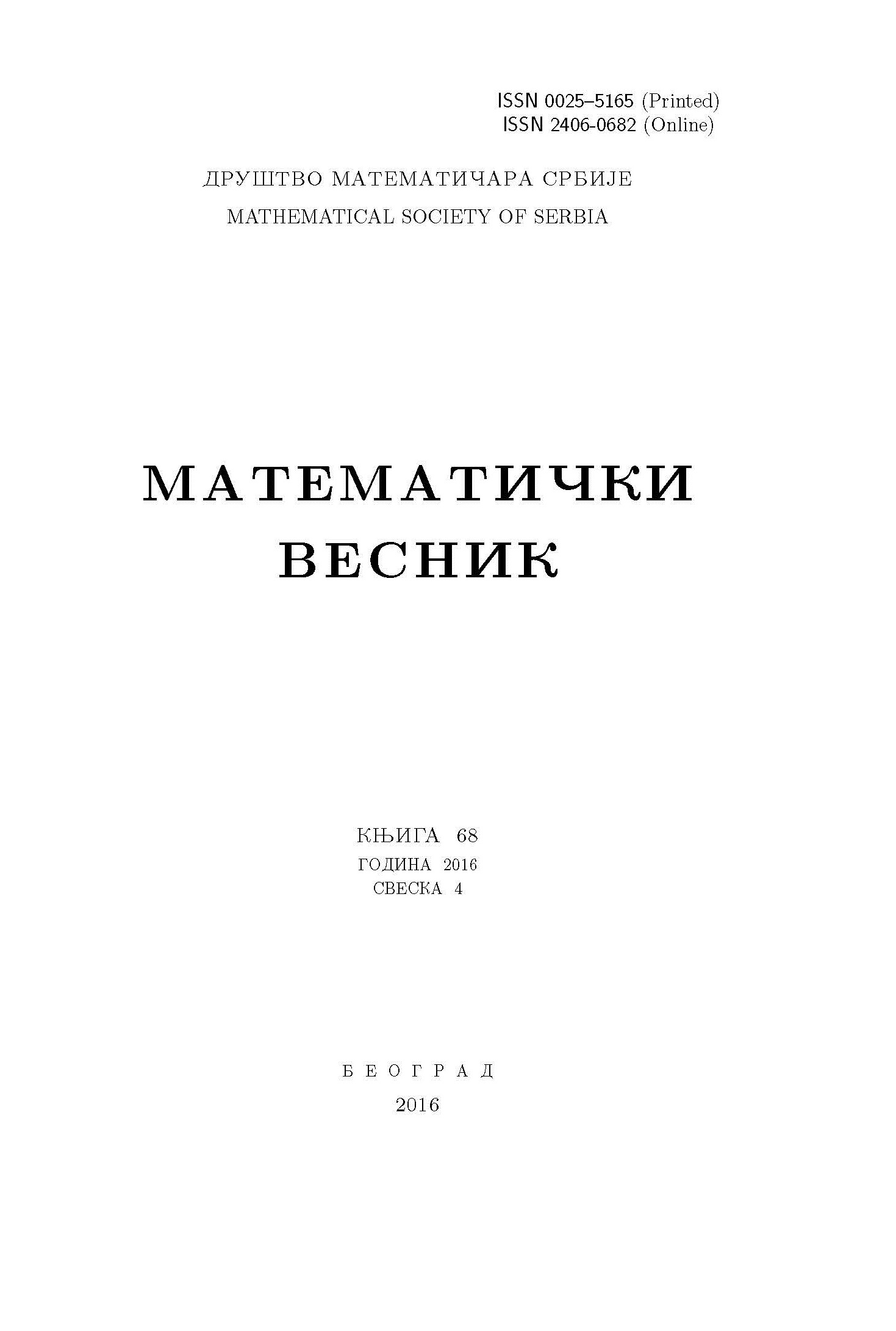﻿ Matematički Vesnik ﻿
 MATEMATIČKI VESNIK МАТЕМАТИЧКИ ВЕСНИКTHE $\sigma$-POINT-FINITE $cn$-NETWORKS ($ck$-NETWORKS) OF PIXLEY-ROY HYPERSPACES L. Q. Tuyen, O. V. Tuyen AbstractIn this paper, we study the relation between a space $X$ satisfying certain generalized metric properties and the Pixley-Roy hyperspace $\mathcal F[X]$ over $X$ satisfying the same properties. We prove that if $X$ has a $\sigma$-point-finite $cn$-network (resp., $ck$-network), then $\mathcal F[X]$ also has a $\sigma$-point-finite $cn$-network (resp., $ck$-network).Keywords: Pixley-Roy; hyperspace; $cn$-network; $ck$-network; $\sigma$-point-finite. MSC: 54B20, 54D20 Pages:  1$-$4

﻿